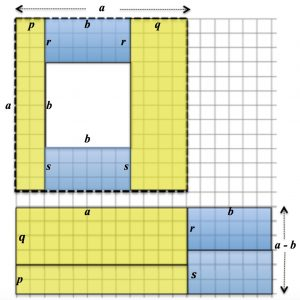1. What do you see in this picture?

Give a proof linked to this picture of the formula $a^2 – b^2 = (a + b)(a – b)$ .

2. What do you notice about the difference between squares of consecutive numbers?

Compare the differences between squares of numbers that differ by 1 such as  $4^2-3^2=7$ with squares of numbers that differ by 2 such as  $5^2-3^2=16$ and $6^2-4^2=20$ . What about squares of numbers that differ by 3, or 4, or 5 ….?

Investigate which numbers can, and which numbers cannot, be written as the difference of two squares and when this can be done in more than one way.  It might help you to make some conjectures about what happens in general if you try to find ways of writing the numbers from 1 to 30 as the difference of two squares.

The big challenge is to prove your conjectures.

Thank you to NRICH for some of the ideas here.  For more challenges about the differences of two squares see Hollow Squares and Plus Minus on the NRICH website.

### One Response to Differences of Squares Investigation

1.Thabiso Ketnot says:

Thank you